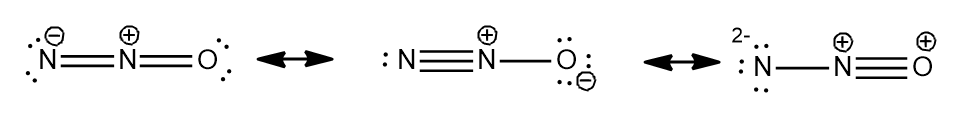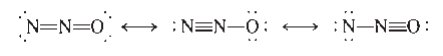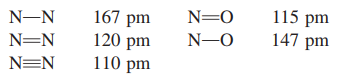# Problem: Nitrous oxide (N2O) has three possible Lewis structures:Given the following bond lengths,rationalize the observations that the N=N bond length in N2O is 112 pm and that the N=O bond length is 119 pm. Assign formal charges to the resonance structures for N2O. Can you eliminate any of the resonance structures on the basis of formal charges? Is this consistent with observation?

###### FREE Expert Solution

We are asked to rationalize the observations that the N=N bond length in N2O is 112 pm and that the N=O bond length is 119 pm.97% (349 ratings)###### Problem Details

Nitrous oxide (N2O) has three possible Lewis structures:Given the following bond lengths,rationalize the observations that the N=N bond length in N2O is 112 pm and that the N=O bond length is 119 pm. Assign formal charges to the resonance structures for N2O. Can you eliminate any of the resonance structures on the basis of formal charges? Is this consistent with observation?

Frequently Asked Questions

What scientific concept do you need to know in order to solve this problem?

Our tutors have indicated that to solve this problem you will need to apply the Average Bond Order concept. You can view video lessons to learn Average Bond Order. Or if you need more Average Bond Order practice, you can also practice Average Bond Order practice problems.

What professor is this problem relevant for?

Based on our data, we think this problem is relevant for Professor Costanza's class at USF.

What textbook is this problem found in?

Our data indicates that this problem or a close variation was asked in Chemistry: An Atoms First Approach - Zumdahl Atoms 1st 2nd Edition. You can also practice Chemistry: An Atoms First Approach - Zumdahl Atoms 1st 2nd Edition practice problems.